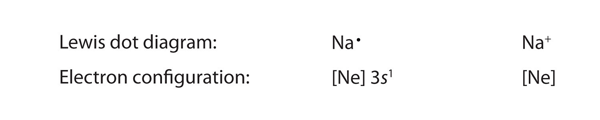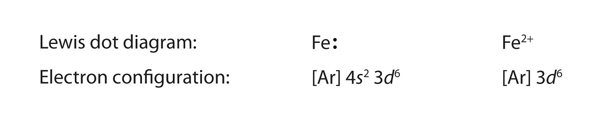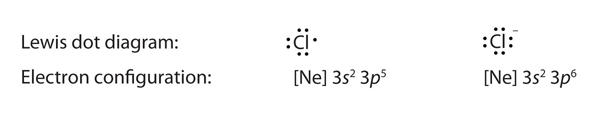Chapter 9. Chemical Bonding and Lewis Structures

# 9.3 Lewis Electron Dot Diagrams

### Learning Objective

By the end of this section, you will be able to:

• Draw a Lewis electron dot diagram for an atom or a monatomic ion.

In almost all cases, chemical bonds are formed by interactions of valence electrons in atoms. To facilitate our understanding of how valence electrons interact, a simple way of representing those valence electrons would be useful.

A Lewis electron dot diagram (or electron dot diagram or a Lewis diagram or a Lewis structure) is a representation of the valence electrons of an atom that uses dots around the symbol of the element. The number of dots equals the number of valence electrons in the atom. These dots are arranged to the right and left and above and below the symbol, with no more than two dots on a side. (It does not matter what order the positions are used.) For example, the Lewis electron dot diagram for hydrogen is simplyBecause the side is not important, the Lewis electron dot diagram could also be drawn as follows:The electron dot diagram for helium, with two valence electrons, is as follows:By putting the two electrons together on the same side, we emphasize the fact that these two electrons are both in the 1s subshell; this is the common convention we will adopt, although there will be exceptions later. The next atom, lithium, has an electron configuration of 1s22s1, so it has only one electron in its valence shell. Its electron dot diagram resembles that of hydrogen, except the symbol for lithium is used:Beryllium has two valence electrons in its 2s shell, so its electron dot diagram is like that of helium:The next atom is boron. Its valence electron shell is 2s22p1, so it has three valence electrons. The third electron will go on another side of the symbol:Again, it does not matter on which sides of the symbol the electron dots are positioned.

For carbon, there are four valence electrons, two in the 2s subshell and two in the 2p subshell. As usual, we will draw two dots together on one side, to represent the 2s electrons. However, conventionally, we draw the dots for the two p electrons on different sides. As such, the electron dot diagram for carbon is as follows:With nitrogen, which has three p electrons, we put a single dot on each of the three remaining sides:For oxygen, which has four p electrons, we now have to start doubling up on the dots on one other side of the symbol. When doubling up electrons, make sure that a side has no more than two electrons.Fluorine and neon have seven and eight dots, respectively:With the next element, sodium, the process starts over with a single electron because sodium has a single electron in its highest-numbered shell, the n = 3 shell. By going through the periodic table, we see that the Lewis electron dot diagrams of atoms will never have more than eight dots around the atomic symbol.

### Example 1

What is the Lewis electron dot diagram for each element?      a) aluminum         b) selenium

Solution

a) The valence electron configuration for aluminum is 3s23p1. So it would have three dots around the symbol for aluminum, two of them paired to represent the 3s electrons:b) The valence electron configuration for selenium is 4s24p4. In the highest-numbered shell, the n = 4 shell, there are six electrons. Its electron dot diagram is as follows:Test Yourself

What is the Lewis electron dot diagram for each element?  a) phosphorus          b) argon

For atoms with partially filled d or f subshells, these electrons are typically omitted from Lewis electron dot diagrams. For example, the electron dot diagram for iron (valence shell configuration 4s23d6) is as follows:Elements in the same column of the periodic table have similar Lewis electron dot diagrams because they have the same valence shell electron configuration. Thus the electron dot diagrams for the first column of elements are as follows:Monatomic ions are atoms that have either lost (for cations) or gained (for anions) electrons. Electron dot diagrams for ions are the same as for atoms, except that some electrons have been removed for cations, while some electrons have been added for anions. Thus in comparing the electron configurations and electron dot diagrams for the Na atom and the Na+ ion, we note that the Na atom has a single valence electron in its Lewis diagram, while the Na+ ion has lost that one valence electron:Technically, the valence shell of the Na+ ion is now the n = 2 shell, which has eight electrons in it. So why do we not put eight dots around Na+? Conventionally, when we show electron dot diagrams for ions, we show the original valence shell of the atom, which in this case is the n = 3 shell and empty in the Na+ ion.

In making cations, electrons are first lost from the highest numbered shell, not necessarily the last subshell filled. For example, in going from the neutral Fe atom to the Fe2+ ion, the Fe atom loses its two 4s electrons first, not its 3d electrons, despite the fact that the 3d subshell is the last subshell being filled. Thus we haveAnions have extra electrons when compared to the original atom. Here is a comparison of the Cl atom with the Cl ion:### Example 2

What is the Lewis electron dot diagram for each ion?   a) Ca2+         b) O2−

Solution

a) Having lost its two original valence electrons, the Lewis electron dot diagram is just Ca2+.

Ca2+

b) The O2− ion has gained two electrons in its valence shell, so its Lewis electron dot diagram is as follows:Test Yourself

The valence electron configuration of thallium, whose symbol is Tl, is 6s25d106p1. What is the Lewis electron dot diagram for the Tl+ ion?

## Key Concepts and Summary

Lewis electron dot diagrams use dots to represent valence electrons around an atomic symbol.   Lewis electron dot diagrams for ions have fewer (for cations) or more (for anions) dots than the corresponding atom.

### Exercises

1. Explain why the first two dots in a Lewis electron dot diagram are drawn on the same side of the atomic symbol.

2. What column of the periodic table has Lewis electron dot diagrams with two electrons?

3. Draw the Lewis electron dot diagram for each element.   a)  strontium      b)  silicon

4. Draw the Lewis electron dot diagram for each element.   a)  titanium        b)  phosphorus

5. Draw the Lewis electron dot diagram for each ion.  a)  Mg2+   b)  S2−

6. Draw the Lewis electron dot diagram for each ion.  a)  Fe2+     b)  N3−

1. The first two electrons in a valence shell are s electrons, which are paired.

2. the second column of the periodic table

5. a)   Mg2+

6. a)  Fe2+